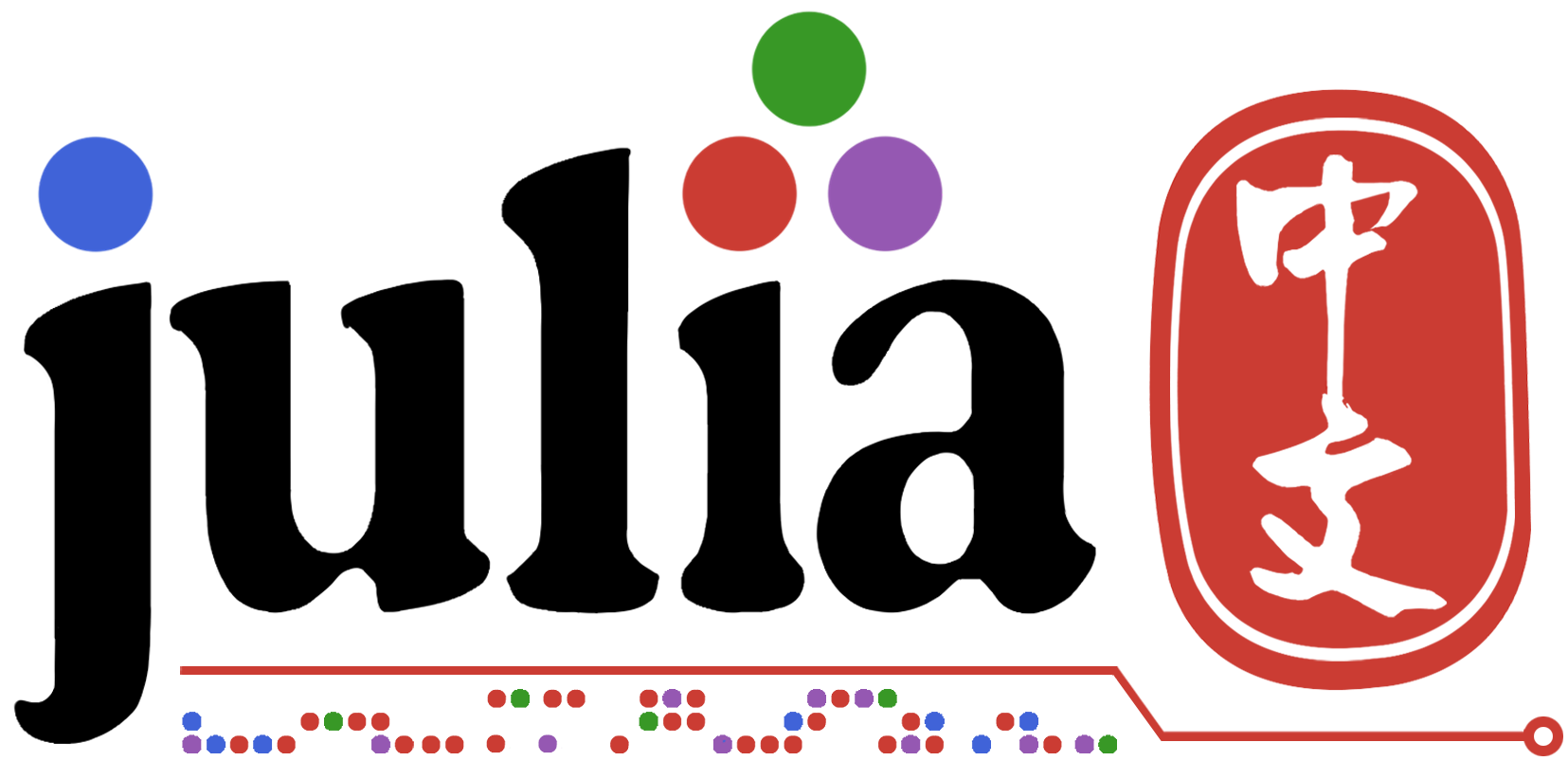# 为啥手动计算的标准差和std()的结果不一样？

``````tl1=[1,2,2,3,3,3,4,4,5]
tl2=tl1

sum_t1=sum(tl1)
meam_t1=sum_t1/length(tl1)

for i=1:length(tl1)
tl2[i]=(tl1[i]-meam_t1)^2
end

sum_t2=sum(tl2)
sig=√(sum_t2/length(tl1))

println("手动sig:",sig)

using Statistics

println("sig:",std(tl1))
``````

help里有写`The algorithm returns an estimator of the generative distribution's standard deviation under the assumption that each entry of itr is an IID drawn from that generative distribution.`

``````julia> itr=[1,2,2,3,3,3,4,4,5]
...

julia> sqrt(sum((itr .- mean(itr)).^2) / (length(itr) - 1))
1.224744871391589

julia> std(itr)
1.224744871391589

julia> sqrt(sum((itr .- mean(itr)).^2) / length(itr))
1.1547005383792515

julia> std(itr,corrected=false)
1.1547005383792515
``````